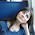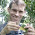Sunday, January 29, 2012

New version of plotSimmap that can plot node numbers

I just posted a new version of plotSimmap (direct link to code here) which can also plot node numbers (that is, the numbers in the matrix \$edge for all non-terminal nodes). These are the same labels used by nodelabels in ape if no node names have been assigned.

This was pretty easy. I just plotted a rectangle with white background (using the graphics function symbols) at the end of each branch not ending in a tip. (I have to figure out where these branches go anyway, so the problem of identifying the vertical position is already solved.) I specified the height & width of box to be slightly larger than the label text length & width using strheight and strwidth. I then wrote inside the box using text.

The result can be visualized by doing something like the following:

require(phytools)
source("plotSimmap.R") # load the new version of plotSimmap
Q<-matrix(c(-1,1,1,-1),2,2)
cols<-c("blue","red"); names(cols)<-c(1,2)
tree<-sim.history(pbtree(n=25,scale=1),Q,anc=1)
plotSimmap(tree,cols,lwd=3,pts=F,node.numbers=T)That's pretty cool. Since plotTree is now just a wrapper for plotSimmap with a really simple change to plotTree (code here) you can now visualize node numbers using that function as well (although this is pretty much completely redundant with plot.phylo and nodelabels in ape).

source("plotTree.R") # load the new version of plotTree
plotTree(tree,pts=F,lwd=3,node.numbers=T)That's it!

1.Thanks a lot for a very nice plotting function (and for a great blog)!

I was wondering: is it possible to use nodelabels(pie=data,cex=0.4) from ape to draw piecharts on top of the plotSimmap (at nodes)?

I've tried a bit with the default settings, but it didn't do a good job with placing piecharts at correct nodes. Changing margins didn't help too.

1.This is possible, but difficult. plotSimmap & plot.phylo do not naturally use the same coordinate system. I will post about this today. - Liam

2.Here is one solution!

Note: due to the very large amount of spam, all comments are now automatically submitted for moderation.